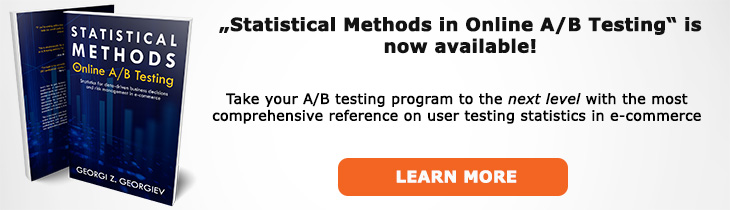# What does "Mean Square Error" mean?

Definition of Mean Square Error in the context of A/B testing (online controlled experiments).

## What is Mean Square Error?

Aliases: MSE

The mean square error (MSE) is a measure of optimality of a statistical estimator. There are two conflicting definitions for optimality in the two major inferential schools: frequentist and Bayesian .

In frequentist inference an efficient estimator (optimal estimator) deviates as little as possible from the true value (θ*) one is trying to estimate: MSE(θ̂n(X);θ*) = E(θ̂n(X) - θ*)2. Thus, the mean square error is defined with respect to the true state of nature, the true underlying data-generating mechanism (DGM) which in hypothesis testing is specified by defining a statistical model.

The importance of having frequentist statistics with low mean square error is then a natural extension of our desire to have an accurate estimation of the true data-generating mechanism. This then translates into making as informed decisions following an A/B test as possible.

In Bayesian inference the decision-theoretic definition of optimiality is used in which an optimal estimator is one that minimizes the loss function over every possible value of the parameter: MSE1(θ̂(X);θ)= E(θ̂(X) - θ)2 = R(θ,θ̂),∀θ∈Θ where R is a risk function. Unlike the frequentist notion, the Bayesian one has no reference to the true value of θ (θ*) and is in fact in direct contrast to the goal of minimizing the error with respect to it and of learning about the true data-generating mechanism. Instead, the objective is to minimize losses weighted by π(θ|x0) the posterior distribution of θ given x0 for all values of θ in Θ.

References:
 Spanos A. (2017) "Why the Decision-Theoretic Perspective Misrepresents Frequentist Inference - Revisiting Steins Paradox and Admissibility"

## Related A/B Testing terms

Variance

Efficient Estimator

Estimator

Estimation

Like this glossary entry? For an in-depth and comprehensive reading on A/B testing stats, check out the book "Statistical Methods in Online A/B Testing" by the author of this glossary, Georgi Georgiev.## Glossary Index by Letter

Select a letter to see all A/B testing terms starting with that letter or visit the Glossary homepage to see all.